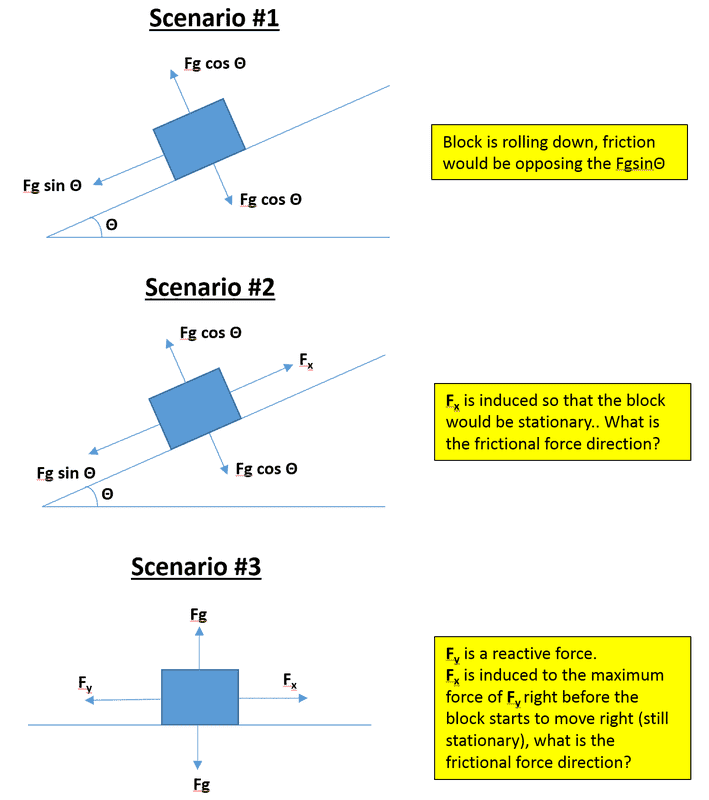# Direction of Frictional Forces on Balanced Force Objects

• physicsnoob204

#### physicsnoob204

TL;DR Summary
What is the direction of the frictional force in these 3 scenarios?
What is the direction of the frictional forces especially when there are 2 forces acting on an object? (see attached Image)1) if the object moves then frictional force is directed against the velocity
2) if the object does not move then the frictional force is found from equations of equilibrium

Summary:: What is the direction of the frictional force in these 3 scenarios?

What is the direction of the frictional forces especially when there are 2 forces acting on an object? (see attached Image)View attachment 264664
The basic approach is to generalize the equations based on FBD and then solve it.
1.In the First scenario the Frictional force will act in the just opposite to FgsinΘ
2. In the second scenario the Frictional force will act in the direction of Fx as the block is acted by gravitational force and is still in rest . Therefore friction force and Fx are acting opposite to FgsinΘ and preventing the motion of the block.
3.In the third scenario the Frictional force will act in the direction of Fy as Fx is intended to cause the motion.
By the way the FBD is wrong in the third scenario as in the free body diagram only forces which acts on the body is considered. Not those forces which are acted by the body hence the statement that Fy is a reactive force is wrong.